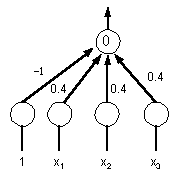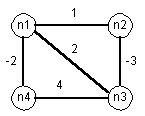## CSC 550/650: Assignment 5

### Expert Systems & Neural Nets

1. Consider the Prolog implementation of a car diagnosis expert system discussed in class (souce code in shell.pro and autoKB.pro).

1. Suppose the following symptoms have been noted (with corresponding certainty factors).
The car starts half the time. (CF = 50)
There appears to be gas in the carburator. (CF = 75)
The plugs do not look very dirty. (CF 20)
The car was tuned fairly recently. (CF = 70)

In addition, the car rides pretty rough, but the user is not quite sure what CF to assign to this symptom. Is there a borderline CF for this symptom? That is, is there a CF that allows for a diagnosis, and yet CF's below (or possibly above) would not? If so, identify the borderline value and explain why the system behaves this way. If not, explain why the CF for this symptom is not relevant.

2. Does the order in which the rules are listed in the knowledge base (autoKB.pro) affect the diagnosis? That is, is it possible that changing the order of the clauses might cause a different diagnosis for the same symptoms? If so, give such an example and explain why this occurs. If not, explain why order is unimportant.

2. Consider the following (normalized) perceptron, with 3 input nodes and a single output node.1. What function is computed by this perceptron? That is, for what input patterns does this perceptron fire?

2. Similar to the examples from class, train this perceptron (with initial weights as shown here) to compute the OR function on three inputs. When the final weights are determined, the perceptron should fire as long as any of the three inputs is a 1. Be sure to intermediate steps in going from the initial weights to the final weights.

3. Consider the Hopfield net given by the following diagram.1. What are the stable states of this network? That is, for which states does parallel relaxation make no changes to the active/inactive status of any nodes?

2. Assume that the nodes labeled n2 and n4 are active and the other two states are inactive. To which stable state would parallel relaxation lead from here? Is it possible that more than one stable state might be reached, depending upon the order in which nodes are selected?

3. Assume that only the node labeled n1 is active and the other three states are inactive. To which stable state would parallel relaxation lead from here? Is it possible that more than one stable state might be reached, depending upon the order in which nodes are selected?